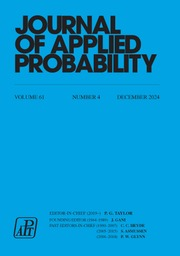Home
Hostname: page-component-6c8bd87754-4q2hw Total loading time: 0.19 Render date: 2022-01-16T23:26:47.216Z Has data issue: true Feature Flags: { "shouldUseShareProductTool": true, "shouldUseHypothesis": true, "isUnsiloEnabled": true, "metricsAbstractViews": false, "figures": true, "newCiteModal": false, "newCitedByModal": true, "newEcommerce": true, "newUsageEvents": true }Journal of Applied Probability

Inhomogeneous random graphs, isolated vertices, and Poisson approximation

Published online by Cambridge University Press:  28 March 2018

Abstract

Consider a graph on randomly scattered points in an arbitrary space, with any two points x, y connected with probability ϕ(x, y). Suppose the number of points is large but the mean number of isolated points is O(1). We give general criteria for the latter to be approximately Poisson distributed. More generally, we consider the number of vertices of fixed degree, the number of components of fixed order, and the number of edges. We use a general result on Poisson approximation by Stein's method for a set of points selected from a Poisson point process. This method also gives a good Poisson approximation for U-statistics of a Poisson process.

MSC classification

Type
Research Papers
Information
Journal of Applied Probability , March 2018 , pp. 112 - 136

Access options

Get access to the full version of this content by using one of the access options below. (Log in options will check for institutional or personal access. Content may require purchase if you do not have access.)

References

Barbour, A. D., Holst, L. and Janson, S. (1992). Poisson Approximation (Oxford Studies Prob. 2). Oxford University Press. Google Scholar
Bhamidi, S., van der Hofstad, R. and van Leeuwaarden, J. S. H. (2012). Novel scaling limits for critical inhomogeneous random graphs. Ann. Prob. 40, 22992361. CrossRefGoogle Scholar
Bollobás, B. (2001). Random Graphs (Camb. Studies Adv. Math. 73), 2nd edn. Cambridge University Press. CrossRefGoogle Scholar
Bollobás, B., Janson, S. and Riordan, O. (2007). The phase transition in inhomogeneous random graphs. Random Structures Algorithms 31, 3122. CrossRefGoogle Scholar
Decreusefond, L., Schulte, M. and Thäle, C. (2016). Functional Poisson approximation in Kantorovich-Rubinstein distance with applications to U-statistics and stochastic geometry. Ann. Prob. 44, 21472197. CrossRefGoogle Scholar
Devroye, L. and Fraiman, N. (2014). Connectivity of inhomogeneous random graphs. Random Structures Algorithms 45, 408420. CrossRefGoogle Scholar
Díaz, J., Petit, J. and Serna, M. (2000). Faulty random geometric networks. Parallel Process. Lett. 10, 343357. CrossRefGoogle Scholar
Franceschetti, M. and Meester, R. (2007). Random Networks for Communication. Cambridge University Press. Google Scholar
Gupta, B. and Iyer, S. K. (2010). Criticality of the exponential rate of decay for the largest nearest-neighbor link in random geometric graphs. Adv. Appl. Prob. 42, 631658. CrossRefGoogle Scholar
Gupta, P. and Kumar, P. R. (1999). Critical power for asymptotic connectivity in wireless networks. In Stochastic Analysis, Control, Optimization and Applications, Birkhäuser, Boston, MA, pp. 547566. CrossRefGoogle Scholar
Hoff, P. D., Raftery, A. E. and Handcock, M. S. (2002). Latent space approaches to social network analysis. J. Amer. Statist. Assoc. 97, 10901098. CrossRefGoogle Scholar
Hsing, T. and Rootzén, H. (2005). Extremes on trees. Ann. Prob. 33, 413444. CrossRefGoogle Scholar
Iyer, S. K. and Thacker, D. (2012). Nonuniform random geometric graphs with location-dependent radii. Ann. Appl. Prob. 22, 20482066. CrossRefGoogle Scholar
Kingman, J. F. C. (1993). Poisson Processes (Oxford Studies Prob. 3). Oxford University Press. Google Scholar
Last, G. and Penrose, M. (2018). Lectures on the Poisson Process (IMS Textbooks 7). Cambridge University Press. Google Scholar
Lindvall, T. (1992). Lectures on the Coupling Method. John Wiley, New York. Google Scholar
Mao, G. and Anderson, B. D. O. (2012). Towards a better understanding of large-scale network models. IEEE/ACM Trans. Networking 20, 408421. CrossRefGoogle Scholar
Meester, R. and Roy, R. (1996). Continuum Percolation (Camb. Tracts Math. 119). Cambridge University Press. CrossRefGoogle Scholar
Penrose, M. D. (1998). Extremes for the minimal spanning tree on normally distributed points. Adv. Appl. Prob. 30, 628639. CrossRefGoogle Scholar
Penrose, M. D. (1999). On k-connectivity for a geometric random graph. Random Structures Algorithms 15, 145164. 3.0.CO;2-G>CrossRefGoogle Scholar
Penrose, M. (2003). Random Geometric Graphs (Oxford Studies Prob. 5). Oxford University Press. CrossRefGoogle Scholar
Penrose, M. D. (2016). Connectivity of soft random geometric graphs. Ann. Appl. Prob. 26, 9861028. CrossRefGoogle Scholar
Rastelli, R., Friel, N. and Raftery, A. E. (2016). Properties of latent variable network models. Network Sci. 4, 407432. CrossRefGoogle Scholar
Snijders, T. A. B. and Nowicki, K. (1997). Estimation and prediction for stochastic blockmodels for graphs with latent block structure. J. Classification 14, 75100. CrossRefGoogle Scholar
Söderberg, B. (2002). General formalism for inhomogeneous random graphs. Phys Rev. E 66, 066121. CrossRefGoogle ScholarPubMed
Yi, C.-W., Wan, P.-J., Lin, K.-W. and Huang, C.-H. (2006). Asymptotic distribution of the number of isolated nodes in wireless ad hoc networks with unreliable nodes and links. In Proc. Global Telecommun. Conf., 2006, IEEE. Google Scholar
6
Cited by

Send article to Kindle

Note you can select to send to either the @free.kindle.com or @kindle.com variations. ‘@free.kindle.com’ emails are free but can only be sent to your device when it is connected to wi-fi. ‘@kindle.com’ emails can be delivered even when you are not connected to wi-fi, but note that service fees apply.

Find out more about the Kindle Personal Document Service.

Inhomogeneous random graphs, isolated vertices, and Poisson approximation
Available formats
×

Send article to Dropbox

To send this article to your Dropbox account, please select one or more formats and confirm that you agree to abide by our usage policies. If this is the first time you use this feature, you will be asked to authorise Cambridge Core to connect with your <service> account. Find out more about sending content to Dropbox.

Inhomogeneous random graphs, isolated vertices, and Poisson approximation
Available formats
×

To send this article to your Google Drive account, please select one or more formats and confirm that you agree to abide by our usage policies. If this is the first time you use this feature, you will be asked to authorise Cambridge Core to connect with your <service> account. Find out more about sending content to Google Drive.

Inhomogeneous random graphs, isolated vertices, and Poisson approximation
Available formats
×
×